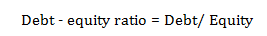# Debt to equity ratio – Definition & Formula

Debt to equity ratio is one of the important ratios, indicating the solvency of a firm. This ratio represents the relationship between borrowed funds (Debt) and owners’ capital (Equity) used by a firm. The ratio is used to measure the long term financial position of a business enterprise.

### Formula for calculating debt to equity ratio:

The given formula can be used for this purpose:### Ideal ratio:

Experts of finance suggest that a company should neither totally rely on equity nor on debt. But there should be an appropriate amount of balance between these two. It would be better for a company to maintain a balance of 2:1 for this ratio. In other words, a company needs to use as much as double the debt than equity.

Because this ratio is used to check the balance between external (Borrower’s) and  internal (Owner’s) fund, it is also known as ‘external- internal ratio’.

Posted on Categories Dictionary